# The DISCRIM Procedure

### Posterior Probability Error-Rate Estimates

The posterior probability error-rate estimates (Fukunaga and Kessel 1973; Glick 1978; Hora and Wilcox 1982) for each group are based on the posterior probabilities of the observations classified into that same group.

A sample of observations with classification results can be used to estimate the posterior error rates. The following notation is used to describe the sample: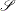the set of observations in the (training) sample

n

the number of observations in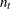the number of observations inin group t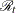the set of observations such that the posterior probability belonging to group t is the largest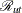the set of observations from group u such that the posterior probability belonging to group t is the largest

The classification error rate for group t is defined as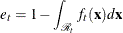The posterior probability offor group t can be written as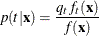where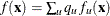is the unconditional density of.

Thus, if you replace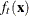with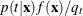, the error rate is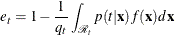An estimator of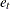, unstratified over the groups from which the observations come, is then given by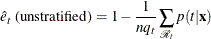where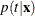is estimated from the classification criterion, and the summation is over all sample observations ofclassified into group t. The true group membership of each observation is not required in the estimation. The term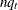is the number of observations that are expected to be classified into group t, given the priors. If more observations than expected are classified into group t, then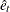can be negative.

Further, if you replace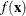with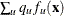, the error rate can be written as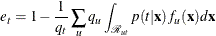and an estimator stratified over the group from which the observations come is given by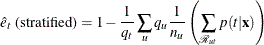The inner summation is over all sample observations ofcoming from group u and classified into group t, and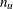is the number of observations originally from group u. The stratified estimate uses only the observations with known group membership. When the prior probabilities of the group membership are proportional to the group sizes, the stratified estimate is the same as the unstratified estimator.

The estimated group-specific error rates can be less than zero, usually due to a large discrepancy between prior probabilities of group membership and group sizes. To have a reliable estimate for group-specific error rate estimates, you should use group sizes that are at least approximately proportional to the prior probabilities of group membership.

A total error rate is defined as a weighted average of the individual group error rates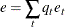and can be estimated from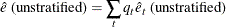or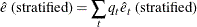The total unstratified error-rate estimate can also be written as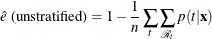which is one minus the average value of the maximum posterior probabilities for each observation in the sample. The prior probabilities of group membership do not appear explicitly in this overall estimate.